# Week 3 Values and Types Types of values

• Slides: 17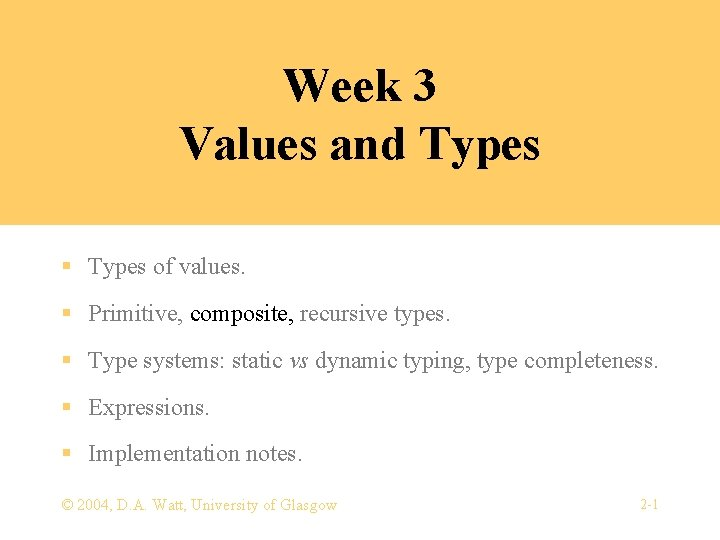Week 3 Values and Types § Types of values. § Primitive, composite, recursive types. § Type systems: static vs dynamic typing, type completeness. § Expressions. § Implementation notes. © 2004, D. A. Watt, University of Glasgow 2 -1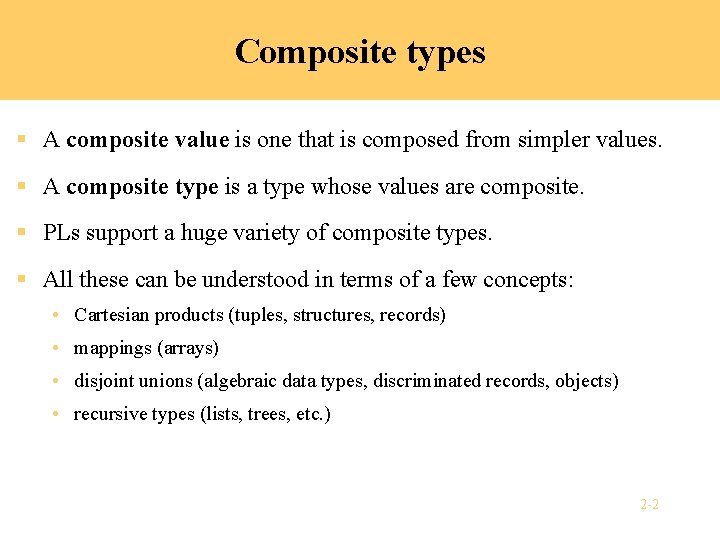Composite types § A composite value is one that is composed from simpler values. § A composite type is a type whose values are composite. § PLs support a huge variety of composite types. § All these can be understood in terms of a few concepts: • Cartesian products (tuples, structures, records) • mappings (arrays) • disjoint unions (algebraic data types, discriminated records, objects) • recursive types (lists, trees, etc. ) 2 -2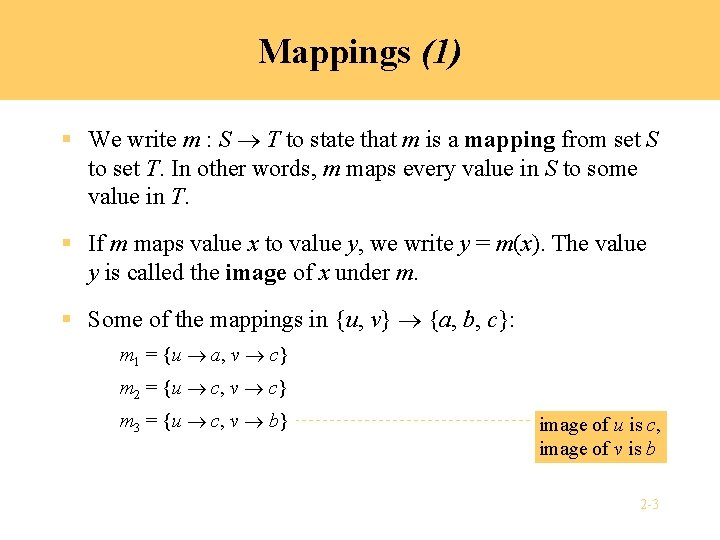Mappings (1) § We write m : S T to state that m is a mapping from set S to set T. In other words, m maps every value in S to some value in T. § If m maps value x to value y, we write y = m(x). The value y is called the image of x under m. § Some of the mappings in {u, v} {a, b, c}: m 1 = {u a, v c} m 2 = {u c, v c} m 3 = {u c, v b} image of u is c, image of v is b 2 -3Mappings (2) § Let S T stand for the set of all mappings from S to T: S T = { m | x Î S Þ m(x) Î T } § What is the cardinality of S T? There are #S values in S. Each value in S has #T possible images under a mapping in S T. So there are #T … #T possible mappings. Thus: #(S T) = (#T)#S #S copies of #T multiplied together § For example, in {u, v} {a, b, c} there are 32 = 9 possible mappings. 2 -4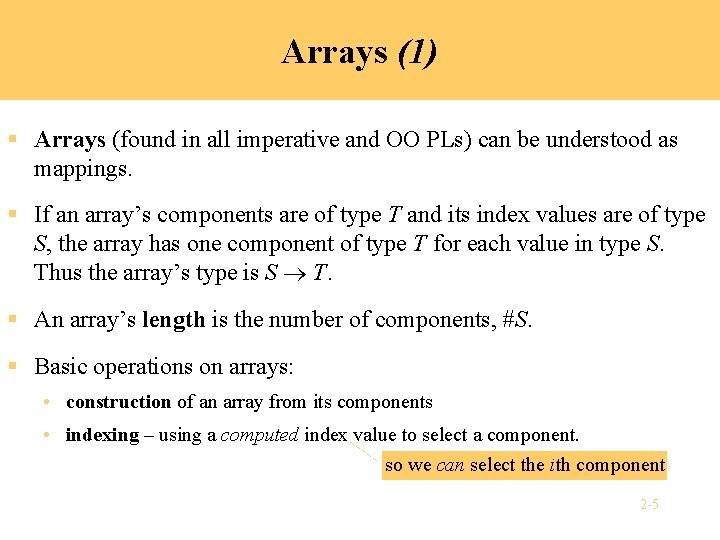Arrays (1) § Arrays (found in all imperative and OO PLs) can be understood as mappings. § If an array’s components are of type T and its index values are of type S, the array has one component of type T for each value in type S. Thus the array’s type is S T. § An array’s length is the number of components, #S. § Basic operations on arrays: • construction of an array from its components • indexing – using a computed index value to select a component. so we can select the ith component 2 -5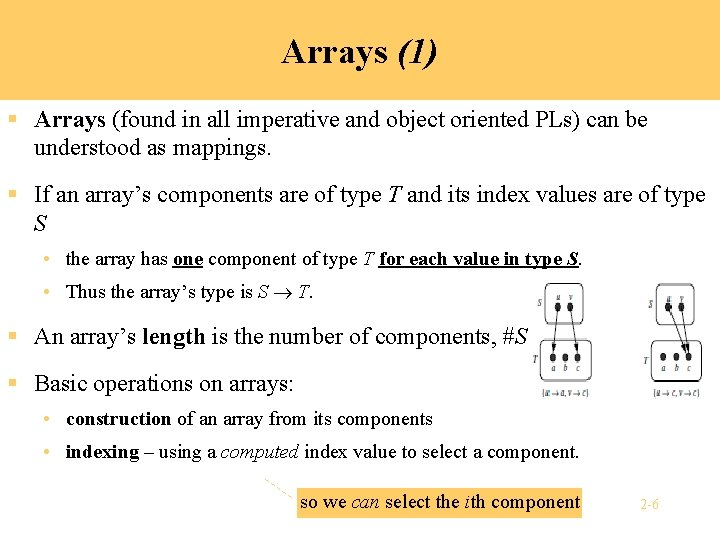Arrays (1) § Arrays (found in all imperative and object oriented PLs) can be understood as mappings. § If an array’s components are of type T and its index values are of type S • the array has one component of type T for each value in type S. • Thus the array’s type is S T. § An array’s length is the number of components, #S. § Basic operations on arrays: • construction of an array from its components • indexing – using a computed index value to select a component. so we can select the ith component 2 -6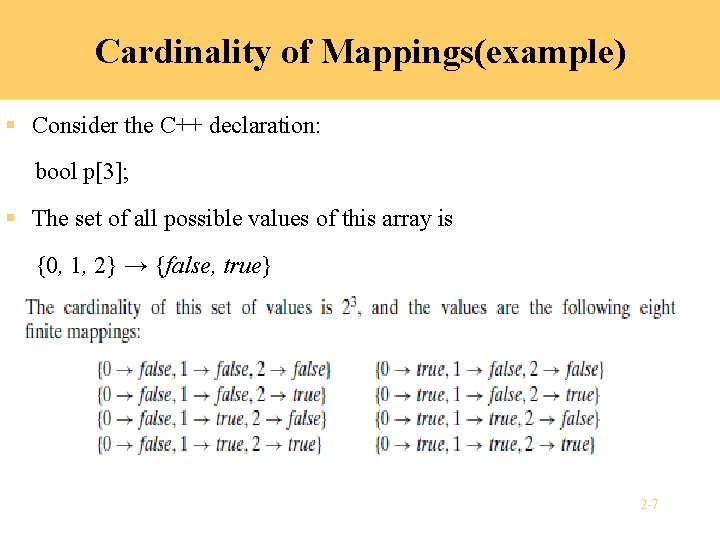Cardinality of Mappings(example) § Consider the C++ declaration: bool p; § The set of all possible values of this array is {0, 1, 2} → {false, true} 2 -7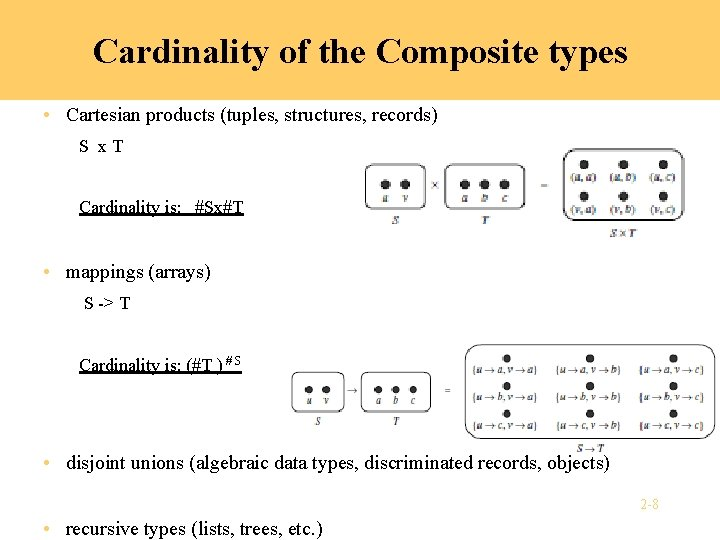Cardinality of the Composite types • Cartesian products (tuples, structures, records) S x. T Cardinality is: #Sx#T • mappings (arrays) S -> T Cardinality is: (#T ) # S • disjoint unions (algebraic data types, discriminated records, objects) 2 -8 • recursive types (lists, trees, etc. )Arrays (2) § An array of type S T is a finite mapping. § Here S is nearly always a finite range of consecutive values {l, l+1, …, u}. This is called the array’s index range. lower bound upper bound § In C and Java, the index range must be integers {0, 1, …, n– 1}. § How about multi-dimensional arrays? • If an array has index ranges of types S 1, …, Sn, the array’s type is S 1 … Sn T. 2 -9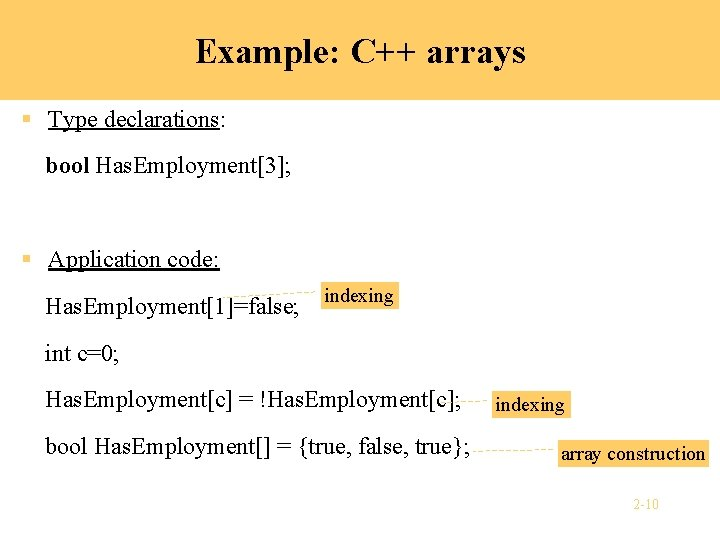Example: C++ arrays § Type declarations: bool Has. Employment; § Application code: Has. Employment=false; indexing int c=0; Has. Employment[c] = !Has. Employment[c]; bool Has. Employment[] = {true, false, true}; indexing array construction 2 -10Example: Perl arrays § No type declarations in Perl and Python! § Perl’s Array construction: @mycolors = (‘red’, ‘green’, ‘blue’); § \$mycolors = ‘blue’; array construction indexing 2 -11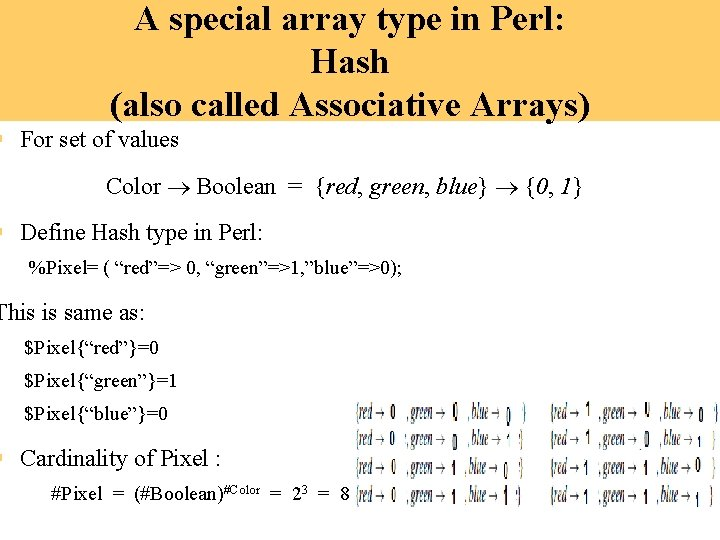A special array type in Perl: Hash (also called Associative Arrays) § For set of values Color Boolean = {red, green, blue} {0, 1} § Define Hash type in Perl: %Pixel= ( “red”=> 0, “green”=>1, ”blue”=>0); This is same as: \$Pixel{“red”}=0 \$Pixel{“green”}=1 \$Pixel{“blue”}=0 § Cardinality of Pixel : #Pixel = (#Boolean)#Color = 23 = 8 2 -12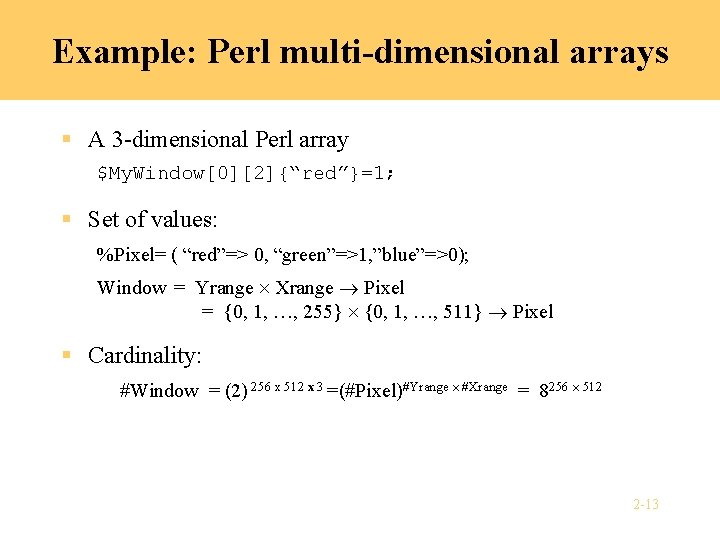Example: Perl multi-dimensional arrays § A 3 -dimensional Perl array \$My. Window{“red”}=1; § Set of values: %Pixel= ( “red”=> 0, “green”=>1, ”blue”=>0); Window = Yrange Xrange Pixel = {0, 1, …, 255} {0, 1, …, 511} Pixel § Cardinality: #Window = (2) 256 x 512 x 3 =(#Pixel)#Yrange #Xrange = 8256 512 2 -13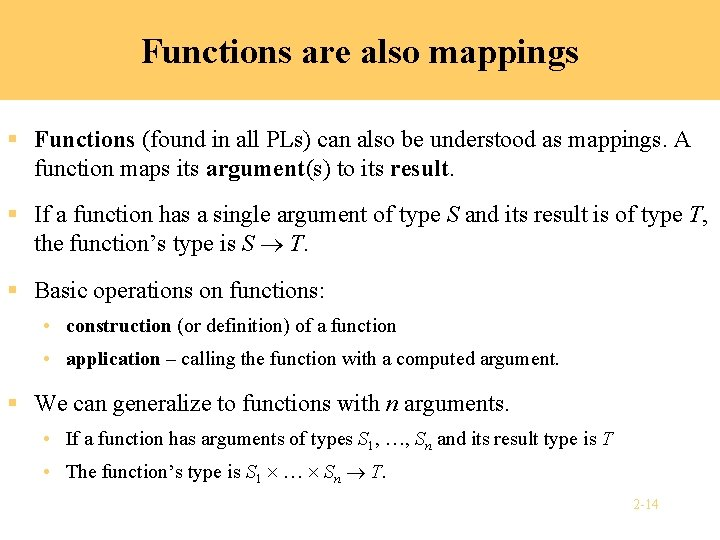Functions are also mappings § Functions (found in all PLs) can also be understood as mappings. A function maps its argument(s) to its result. § If a function has a single argument of type S and its result is of type T, the function’s type is S T. § Basic operations on functions: • construction (or definition) of a function • application – calling the function with a computed argument. § We can generalize to functions with n arguments. • If a function has arguments of types S 1, …, Sn and its result type is T • The function’s type is S 1 … Sn T. 2 -14Mathematical Function or Function Procedure? § A function procedure that inspects or modifies global variables does not correspond to any mathematical function. • i. e. a function procedure that returns the current time of day – this is function procedure • i. e. a function that computes and returns a random number – this is mathematical function § When using the term function in the context of programming languages, we must be very clear whether • Do we mean a mathematical function (mapping)? • Do we mean a function procedure? 2 -15Example: C++ functions § Definition: bool is. Even (int n) { return (n % 2 == 0); } This function implements one particular mapping in Integer→Boolean, namely: {. . . , 0 → true, 1 → false, 2 → true, 3 → false, . . . } § Type: Integer Boolean § Value: {…, 0 true, 1 false, 2 true, 3 false, …} § Other functions of same type: is. Odd, is. Prime, etc. 2 -16